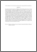# On the estimation of survival function and parameter exponential life time distribution.

## Citation

Al-Kutubi, Hadeel Salim and Ibrahim, Noor Akma (2009) On the estimation of survival function and parameter exponential life time distribution. Journal of Mathematics and Statistics, 5 (2). pp. 130-135. ISSN 1549-3644

## Abstract

The study and research of survival or reliability or life time belong to the same area of study but they may belong to a different area of application. In survival analysis one can use several life time distribution, exponential distribution with mean life time q is one of them. To estimate this parameter and survival function we must be used estimation procedures with less MSE and MPE. Approach: The only statistical theory that combined modeling inherent uncertainty and statistical uncertainty is Bayesian statistics. The theorem of Bayes provided a solution to how learn from data. Bayes theorem was depending on prior and posterior distribution and standard Bayes estimator depends on Jeffery prior information. In this study we annexed Jeffery prior information to get the modify Bayes estimator and then compared it with standard Bayes estimator and maximum likelihood estimator to find the best (less MSE and MPE). Results: when we derived Bayesian and Maximum likelihood of the scale parameter and survival functions. Simulation study was used to compare between estimators and Mean Square Error (MSE) and Mean Percentage Error (MPE) of estimators are computed. Conclusion: The new proposed estimator of modify Bayes estimator in parameter and survival function was the best estimator (less MSE and MPE) when we compared it with standard Bayes and maximum likelihood estimator.Preview
PDF (Abstract)
On the estimation of survival function and parameter exponential life time distribution.pdfView Item